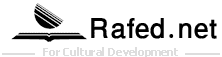## A Mathematical Approach

All of the examples so far given concerning the various angles from which one can approach the Qur'an have undoubtedly been subjective in nature; however, there does exist another angle, among others, which is objective and whose basis is mathematical.

It is surprising how authentic the Qur'an becomes when one assembles what might be referred to as a list of good guesses. Mathematically, it can be explained using guessing and prediction examples. For instance, if a person has two choices (i.e., one is right, and one is wrong), and he closes his eyes and makes a choice, then half of the time (i.e., one time out of two) he will be right. Basically, he has a one in two chance, for he could pick the wrong choice, or he could pick the right choice.

Now if the same person has two situations like that (i.e., he could be right or wrong about situation number one, and he could be right or wrong about situation number two), and he closes his eyes and guesses, then he will only be right one-fourth of the time (i.e., one time out of four). He now has a one in four chance because now there are three ways for him to be wrong and only one way for him to be right. In simple terms, he could make the wrong choice in situation number one and then make the wrong choice in situation number two; or he could make the wrong choice in situation number one and then make the right choice in situation number two; or he could make the right choice in situation number one and then make the wrong choice in situation number two; or he could make the right choice in situation number one and then make the right choice in situation number two.

Of course, the (only instance in which he could be totally right is the last scenario where he could guess correctly in both situations. The odds of his guessing completely correctly have become greater because the number of situations for him to guess in have increased; and the mathematical equation representing such a scenario is ½ x ½ (i.e., one time out of two for the first situation multiplied by one time out of two for the second situation).

Continuing on with the example, if the same person now has three situations in which to make blind guesses, then he will only be right one-eighth of the time (i.e., one time out of eight or ½ x ½ x ½ ). Again, the odds of choosing the correct choice in all three situations have decreased his chances of being completely correct to only one time in eight. It must be understood that as the number of situations increase, the chances of being right decrease, for the two phenomena are inversely proportional.

Now applying this example to the situations in the Qur'an, if one draws up a list of all of the subjects about which the Qur'an has made correct statements, it becomes very clear that it is highly unlikely that they were all just correct blind guesses. Indeed, the subjects discussed in the Qur'an are numerous [some of them are listed in the Qur'an and scientific knowledge, and thus the odds of someone just making lucky guesses about all of them become practically nil. If there are a million ways for the Qur'an to be wrong, yet each time it is right, then it is unlikely that someone was guessing.

Adopted from the book: "The Amazing Qur'an" by: "Gary Miller"

Comment description

### Latest Post

• 2022-08-28 08:09:52 314
• 2021-12-18 20:53:52 447
• 2021-12-11 22:26:45 474

### Most Reviews2022/08/282021/12/182021/12/11